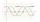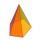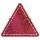# Goniomentry and trigonometry - math word problems

1. CuboidCuboid ABCDEFGH with 10 cm height has a base edge length 6 cm and 8 cm. Determine angle between body diagonal and the base plane (round to degrees).
2. Goat and circlesWhat is the radius of a circle centered on the other circle and the intersection of the two circles is equal to half the area of the first circle? This task is the mathematical expression of the role of agriculture. The farmer has circular land on which
3. BuildingThe building I focused at an angle 30°. When I moved 5 m building I focused at an angle 45°. What is the height of the building?
4. TreeHow tall is the tree that observed in the visual angle of 52°? If I stand 5 m from the tree and eyes are two meters above the ground.
5. AngleA straight line p given by the equation ?. Calculate the size of angle in degrees between line p and y-axis.
6. SinusDetermine the smallest integer p for which the equation 4 sin x = p has no solution.
7. Pentagonal pyramidCalculate the volume of a regular 5-side (pentaprism) pyramid ABCDEV; if |AB| = 7.7 cm and a plane ABV, ABC has angle 37 degrees.
8. Triangular prismPlane passing through the edge AB and the center of segmet CC' of regular triangular prism ABCA'B'C', has angle with base 22 degrees, |AB| = 6 cm. Calculate the volume of the prism.
9. Hexagon 5The distance of parallel sides of regular hexagonal is 61 cm. Calculate the length of the radius of the circle described to this hexagon.
10. CosineThe point (8, 6) is on the terminal side of angle θ. cos θ = ?
11. Vector sumThe magnitude of the vector u is 12 and the magnitude of the vector v is 8. Angle between vectors is 61°. What is the magnitude of the vector u + v?
12. DiagonalsCalculate the length of the diagonals of the rhombus if its side is long 5 and one of its internal angle is 80°.
13. CosineCalculate the cosine of the smallest internal angle in a right-angled triangle with cathetus 3 and 8 and with the hypotenuse 8.544.
14. ISO trapeziumCalculate area of isosceles trapezoid with base 95 long, leg 27 long and with the angle between the base and leg 70 degrees.
15. 30-60-90The longer leg of a 30°-60°-90° triangle measures 5. What is the length of the shorter leg?
16. Angle between vectorsFind the angle between the given vectors to the nearest tenth of a degree. u = (-22, 11) and v = (16, 20)
17. Chord - TSThe radius of circle k measures 68 cm. Arc GH = 47 cm. What is TS?
18. EQL triangleCalculate inradius and circumradius of equilateral triangle with side a=77 cm.
19. FlowerbedFlowerbed has the shape of an isosceles obtuse triangle. Arm has a size 5.5 meters and an angle opposite to the base size is 94°. What is the distance from the base to opposite vertex?
20. Cable car 2Cable car rises at an angle 41° and connects the upper and lower station with an altitude difference of 1175 m. How long is the track of cable car?

Do you have an interesting mathematical word problem that you can't solve it? Submit math problem, and we can try to solve it.

We will send a solution to your e-mail address. Solved examples are also published here. Please enter the e-mail correctly and check whether you don't have a full mailbox.

Please do not submit problems from current active competitions such as Mathematical Olympiad, correspondence seminars etc...

Most natural application of trigonometry and trigonometric functions is a calculation of the triangles. Common and less common calculations of different types of triangles offers our triangle calculator. Word trigonometry comes from Greek and literally means triangle calculation.